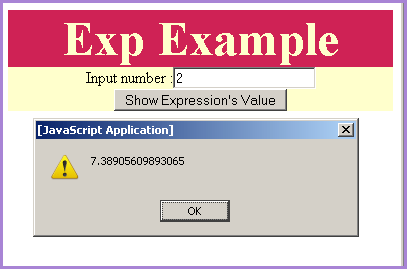Tutorials

JavaScript exp method

We can perform the mathematical calculations in the JavaScript by using the Math object reference.

We can perform the mathematical calculations in the JavaScript by using the Math object reference.

JavaScript exp method

We can perform the mathematical calculations in the JavaScript by using the Math object reference. In Math object we have some very useful functions in which exp() is one important function generally used by the programmers. The exp() method of Math object returns the value of ex , where "e" is the Euler's constant whose value is approximately 2.71828182846. Syntax for using exp() method is as given below:

Syntax:

 Math.exp( number);

Description of Code:

In our following sample code we have created an input text which will take input by the user and when user clicks on the button "Show Expression's Value"  it calls the function callExp() . This function takes the input number by calling document.getElementById() and then we have shown output expression value in the alert() message. Here is the full html code for expExample.html as follows:

expExample.html


Exp Example
Input number :

Output :Input any number to call exp method on it.